This tutorial shows how to run simple radiocarbon models in SoilR. First, we show how to use radiocarbon data to inform a simple one-pool model under the assumption of steady-state. We try to highlight the limitations of this model and its inherent assumptions. In the second part, we show how to run a model not in steady-state by driving the model with time dependent inputs and time varying cycling rates.

Details about the implementation of radiocarbon dynamics in SoilR can be found in Sierra et al. (2014).

First, we run a model under the steady-state assumption and using radiocarbon data to inform the model. Let's assume we have an archived soil sample collected in 1950. The horizon this sample was collected from has a C inventory of 200 gC m-2. The radiocarbon value measured for the bulk sample had a Fraction Modern of 0.749. By a density separation procedure on the sample, and subsequent radiocarbon measurements on the two isolated fractions, we determined that 60% of the carbon has a fraction Modern of 0.9976, and 40% has fraction Modern of 0.3762. We will also use the convention T= bulk sample, A = active pool, P = passive pool.

For implementing this model in SoilR, we open an R session and load the SoilR package:

library(SoilR)


We now create a few objects in R with the information we have (text after the # symbol are comments in the code).

#Data bulk sample
yr=1950
FmT=0.749
Cinv=200

#Data for fractions
FmA=0.9976
fA=0.4
FmB=0.3762
fB=0.6


Under the steady-state assumption we can assume that the decomposition rate is a function of the fraction modern value $$Fm$$ and the radiocarbon decay constant $$\lambda$$. That is $\label{kss} k =\frac{Fm \cdot \lambda}{1 - Fm}.$ This formula allows us to easily estimate the decomposition rate of carbon based only on a radiocarbon value. However, to use this formula one must assume a system in steady-state, with constant inputs and decomposition rates, and with a constant value of atmospheric radiocarbon of Fm = 1. For details about the derivation of this formula see Trumbore et al. (2016).

We can implement this simple function in R to calculate $$k$$ values for the different fraction modern values we have.

lambda=1/8267

k=function(Fm){
(Fm*lambda)/(1-Fm)
}

kT=k(FmT)
kA=k(FmA)
kB=k(FmB)


Notice that we already calculated the value of the decomposition rate for samples T, A, and B. Under steady-state we also assume that the inputs of carbon to the soil can be calculated as $$I = k \cdot C$$, which for the sample T can be calculated as

InT=kT*Cinv


We have now all elements to run a simple one-pool model with SoilR. For this, we use the function OnepModel14, which requires as arguments: a vector of time steps to calculate the numerical solution, the value of $$k$$, the initial amount of C in the simulation with its $$\Delta$$14C value, the amounts of inputs, and an atmospheric radiocarbon curve. The vector of years and the $$\Delta$$14C value, calculated from $$Fm$$ are created as

years=seq(1951,2009)
D14T=Delta14C_from_AbsoluteFractionModern(FmT)


There are different radiocarbon curves in SoilR. For this example we will use the bomb radiocarbon curves of Hua et al. 2013, which contains data for the temperate zone of the norther hemisphere (Zone 2). Now we can create a model object as

Datm=Hua2013\$NHZone2[,1:2]
singlepool=OnepModel14(t=years,k=kT, C0=Cinv,
F0_Delta14C=D14T,
In=InT, inputFc=Datm)


Up to this point we have not run the model yet. We only created a model object that checks all the numbers you provided are consistent. Now, if we want to run the model and calculate the amount of carbon or radiocarbon over time we use specific functions for this purpose. Functions getC and getF14 calculate these two variables from the model object as

Ct=getC(singlepool)
C14t=getF14(singlepool)


The results of the simulation are now stored in the objects Ct and C14t. We can plot the behavior of the radiocarbon values over time with the following code.

plot(Datm,type="l",xlab="Year",ylab="Delta 14C (per mil)",
xlim=c(1940,2010),ylim=c(-550,1000))
lines(years, C14t, col=4)
points(yr,Delta14C_from_AbsoluteFractionModern(FmT))
legend("topright",
c("Delta 14C Atmosphere", "Delta 14C in SOM"),
lty=1,col=c(1,4), lwd=1, bty="n"
)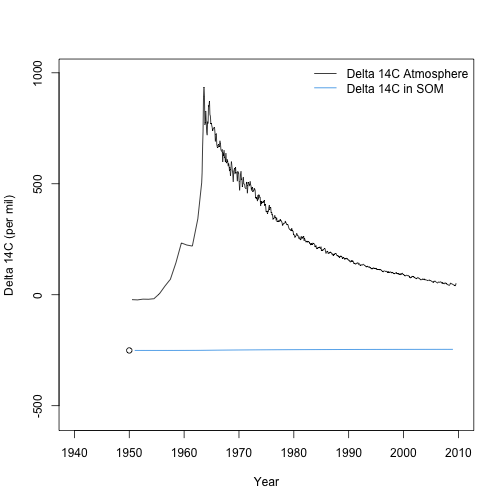The radioactive decay of the sample is imperceptible for this sample given the slow rate $$k$$ calculated from the $$Fm$$ value of the bulk sample

kT

##  0.0003609609


Try now plotting the carbon values over time (Ct); you will see that the system is in steady-state.

We can now run a two pool model using information from samples A and B. The preparatory steps are:

C02=Cinv*c(fA,fB) #Initial amount of C in each fraction
F02=Delta14C_from_AbsoluteFractionModern(c(FmA,FmB)) #Initial Fm for each fraction
I2=(Cinv*fA*kA+Cinv*fB*kB) #Total inputs to soil
gamma=(Cinv*fA*kA)/(Cinv*fA*kA+Cinv*fB*kB) #Proportion of inputs to fast pool


We will use a two-pool model with parallel structure, which is created as

twop=TwopParallelModel14(t=years,ks=c(kA, kB),C0=C02,
F0_Delta14C=F02,In=I2, gam=gamma,
inputFc=Datm)


Running the model and plotting the results gives

C14t2=getF14(twop)

plotC14Pool(t=years,mat=C14t2,inputFc=Datm,col=c(2,4),
ylim=c(-650,1000),ylab="Delta 14 C (per mil)")
points(rep(1950,2),F02)
legend("topright",c("Atmosphere","Pool 1", "Pool 2"),
lty=1,col=c(1,2,4),bty="n")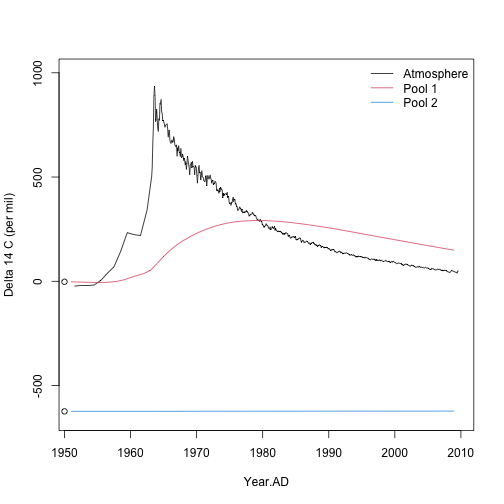This model shows now a more dynamic active pool that incorporates bomb radiocarbon.

Notice that these results are somewhat contradictory. One the one hand, we assumed a constant value of the radiocarbon fraction Fm in our sample to calculate the values of $$k$$. On the other hand, the simulation results show a dynamic radiocarbon pool far from constant, particularly for the active pool. This result points out the problem associate to use radiocarbon data directly to calculate cycling rates or turnover times.

In the previous examples we relied on the assumption of steady-state to run the simulation. However, this is not a requirement in SoilR. In fact, we can run models in which the amount of inputs changes over time, or environmental variables modify the decomposition rates in the model. To run a non-steady-state model however, one must have values of the decomposition rates. For the moment, we will assume that the rates derived in the previous section are appropriate for the non-steady-state case, but this assumption is hardly defensible. One must obtain these values independently or using an optimization procedure.

An artificial dataset of random inputs can be created using the function rnorm, which produces random samples from a normal distribution with certain mean and standard deviation.

randomInputs=data.frame(time=years,In=4+(years-min(years))*0.04+
rnorm(n=length(years),mean=0,sd=0.1))


Similarly, we can create artificial temperature data and use a Q10 function to produce temperature modifiers to decomposition rates

Temp=10+(years-min(years))*0.04+rnorm(n=length(years),mean=0,sd=0.5)
xi=data.frame(years,xi=fT.Q10(Temp,Q10=1.4))


To view these two datasets

par(mfrow=c(2,1), mar=c(5,4,2,2))
plot(randomInputs,type="l", xlab="Years", ylab="Carbon inputs")
plot(xi,type="l", ylab=expression(xi), xlab="Years")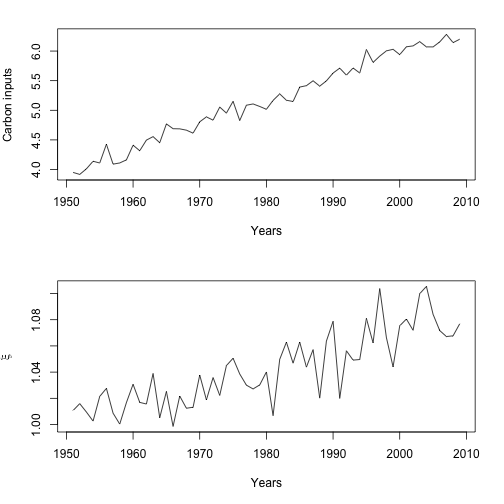par(mfrow=c(1,1))


We can now run a new model with time dependent inputs and variable decomposition rates due to variable temperatures.

twopvar=TwopParallelModel14(t=years,ks=c(kA, kB),C0=C02,
F0_Delta14C=F02,In=randomInputs, gam=gamma,
inputFc=Datm, xi=xi)
C14tvar=getF14(twopvar)
Ctvar=getC(twopvar)


We can compare graphically the behavior of the $$\Delta$$14C values over time. Only small differences can be observed between the two type of models.

plotC14Pool(t=years,mat=C14tvar,inputFc=Datm,col=c(2,4),ylim=c(-650,1000),
ylab="Delta 14 C (per mil)")
points(rep(1950,2),F02)
linesCPool(years,C14t2,col=c(2,4),lty=2)
legend("topright",c("Atmosphere","P1 ss", "P2 ss", "P1 nss", "P2 nss"),
lty=rep(c(1,2),each=2),col=c(1,2,4,2,4),bty="n")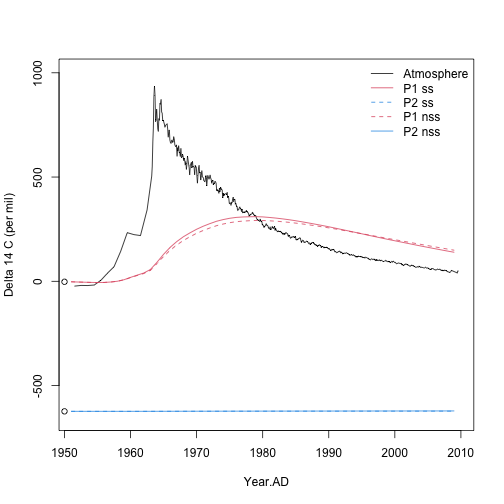However, larger differences can be observed in terms of the carbon stocks in the two pools, particularly for the fast pool.

Ct2=getC(twop)
matplot(years,Ct2,type="l",lty=2,col=c(2,4),ylab="Carbon stocks", xlab="Years", ylim=c(0,200))
linesCPool(years,Ctvar,col=c(2,4),lty=1)
legend("topright",c("P1 ss", "P2 ss", "P1 nss", "P2 nss"),
lty=rep(c(1,2),each=2),col=c(2,4,2,4),bty="n")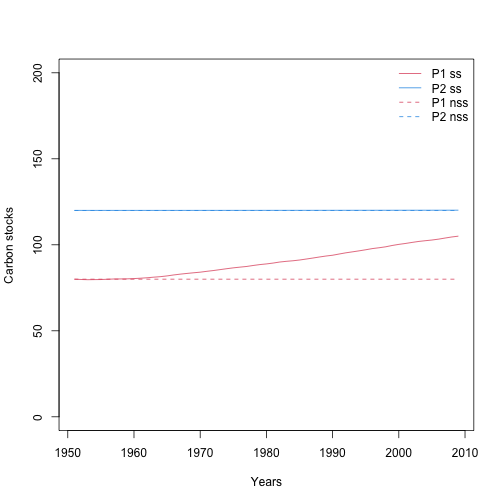## Summary

Running radiocarbon models in SoilR is simple, and they follow the same syntax as any other multiple-pool model. In addition to specifying inputs, decomposition, transfer rates and initial conditions, radiocarbon models must also specify a radiocarbon curve, and the initial radiocarbon value at the beginning of the simulation.

One can use radiocarbon data to infer the decomposition rate of the model assuming the system is well represented by a homogeneous pool in steady-state and constant coefficients, including the radiocarbon atmospheric inputs. In reality these set of assumptions are never met.